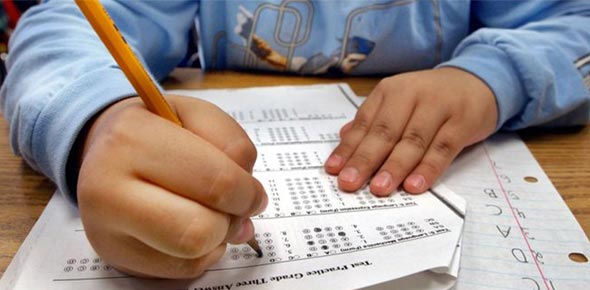# CRCT Grade 2 Math Review

Approved & Edited by ProProfs Editorial Team
The editorial team at ProProfs Quizzes consists of a select group of subject experts, trivia writers, and quiz masters who have authored over 10,000 quizzes taken by more than 100 million users. This team includes our in-house seasoned quiz moderators and subject matter experts. Our editorial experts, spread across the world, are rigorously trained using our comprehensive guidelines to ensure that you receive the highest quality quizzes.
| By Bliss800
B
Bliss800
Community Contributor
Quizzes Created: 7 | Total Attempts: 2,194
Questions: 9 | Attempts: 160SettingsSimple review of math CRCT. These questions are versions of the online CRCT review tests provided by the state of Georgia.

• 1.

### Which number should go in the space?15 + ____ = 17

• A.

5

• B.

27

• C.

2

• D.

12

C. 2
Explanation
The number that should go in the space is 2 because when you subtract 15 from 17, you get 2.

Rate this question:

• 2.

### Courtney wrote down what the other students did during recess.  She put the information in the chart below.GAMES PLAYED AT RECESSEach "X" Stands for 3 students._________________________________________|                                           | Jump Rope          X X X                   ||| Basketball           X X X X   ||| Baseball              X X ||_________________________________________              Remember, each "X" stands for 3 students.QUESTION:How many students played jump rope?

• A.

6

• B.

9

• C.

3

• D.

30

B. 9
Explanation
According to the chart, each "X" stands for 3 students. In the row for Jump Rope, there are 3 "X's" which means that 3 times 3 students played jump rope. Therefore, the total number of students who played jump rope is 9.

Rate this question:

• 3.

### James, Josiah and Sally collected Pokemon cards.They had 9 cards in all.James had 2 cards.Sally had 1 card.How many cards did Josiah have?

• A.

6

• B.

0

• C.

3

• D.

12

A. 6
Explanation
Based on the information given, James had 2 cards and Sally had 1 card. Since the total number of cards collected by James, Josiah, and Sally is 9, and we know that James and Sally had a total of 3 cards, Josiah must have had the remaining 6 cards. Therefore, the correct answer is 6.

Rate this question:

• 4.

### Which number is thirty-four?

• A.

43

• B.

13

• C.

30

• D.

34

D. 34
Explanation
The number that is thirty-four is 34.

Rate this question:

• 5.

### Whic is the expanded form of 4,803?

• A.

48 + 30

• B.

4,000 + 800 + 3

• C.

4000 + 80 + 3

• D.

400 + 80 + 3

• E.

400 + 83

B. 4,000 + 800 + 3
Explanation
The expanded form of 4,803 is 4,000 + 800 + 3. This is because each digit in the number represents its place value. The digit 4 is in the thousands place, so it is multiplied by 4,000. The digit 8 is in the hundreds place, so it is multiplied by 800. The digit 0 is in the tens place, so it is not multiplied by anything. The digit 3 is in the ones place, so it is multiplied by 3. Adding all these products together gives us the expanded form of 4,803.

Rate this question:

• 6.

### Which number is odd?

• A.

0

• B.

342

• C.

17

• D.

68

C. 17
Explanation
The number 17 is odd because it cannot be divided evenly by 2. Odd numbers are those that leave a remainder of 1 when divided by 2. In contrast, even numbers can be divided evenly by 2 without any remainder. Therefore, 17 is the only odd number among the given options.

Rate this question:

• 7.

### Look at the number pattern below: 24  *  27   *   30  *  _____  *  36  *  39  *  42What number belongs in the space?

• A.

32

• B.

31

• C.

33

• D.

19

C. 33
Explanation
The pattern in the number sequence is that each number is obtained by multiplying the previous number by 3 and then adding 3. Starting with 24, we have 24 * 3 + 3 = 27, 27 * 3 + 3 = 30, and so on. Therefore, the missing number should be obtained by multiplying 30 by 3 and then adding 3, which gives us 30 * 3 + 3 = 93.

Rate this question:

• 8.

### Savon went to the store to buy gum.He brought 50 cents.  The gum cost 75 cents.Which number sentence matches this word story?

• A.

\$ .50 + _________ = \$ .75

• B.

\$1 - \$.50 = _________

• C.

\$ .75 + \$ .50 = \$ 1.25

A. \$ .50 + _________ = \$ .75
• 9.

### Three thousand four hundred fifty five What is this number?

• A.

545

• B.

3,405

• C.

3,455

C. 3,455
Explanation
The correct answer is 3,455 because it is the only option that matches the given number "Three thousand four hundred fifty five".

Rate this question:

Related TopicsBack to top